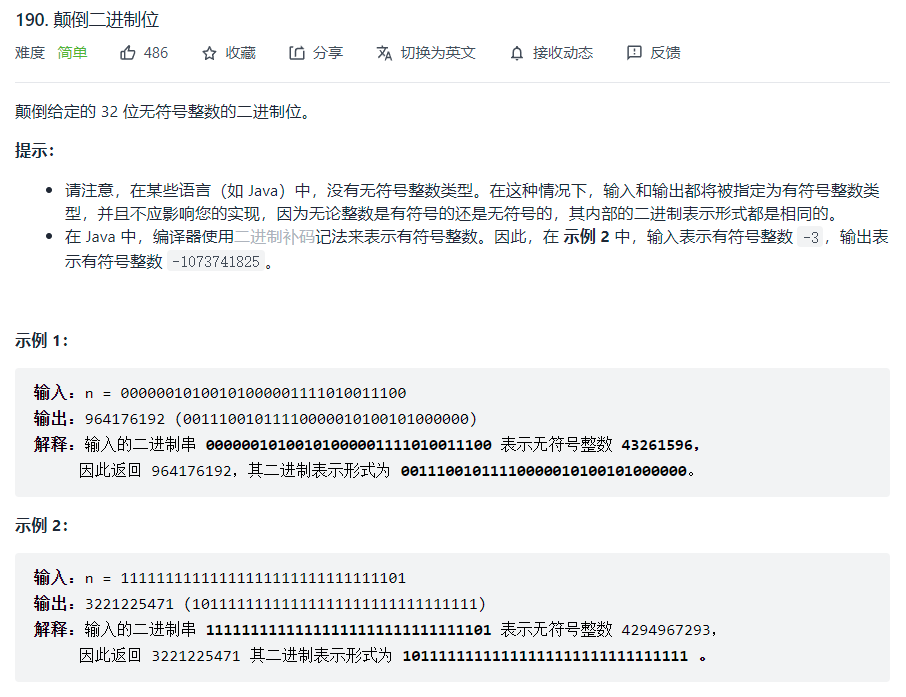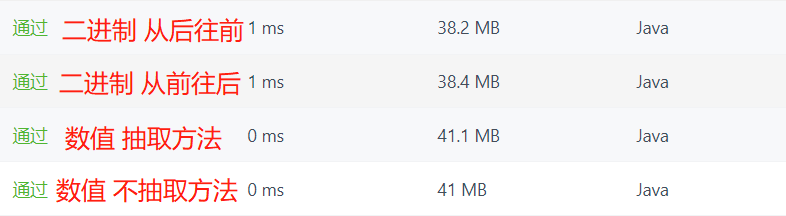# 【LeetCode - Java】190. 颠倒二进制位 （简单）

## 1. 题目描述## 3. 代码实现

### 3.1 数值运算（C语言版）

uint32_t reverseBits(uint32_t n) {
uint32_t sum = 0;
for (int i = 0; i < 32; i++) {
sum *= 2;
sum += n % 2;
n /= 2;
}
return sum;
}### 3.2 数值运算

public int reverseBits(int n) {
if (n < 0) {
n = n << 1;
n = n >>> 1;
return compute(n) + 1;
}
return compute(n);
}

public int compute(int n) {
int sum = 0;
for (int i = 0; i < 32; i++) {
sum *= 2;
sum += n % 2;
n /= 2;
}
return sum;
}### 3.3 二进制运算逐位逆转

public int reverseBits(int n) {
int sum = 0;
for (int i = 0; i < 32; i++) {
// 从前往后
sum += (n << i >>> (31 - i)) & 1 << i;
// 从后往前
//            sum += n & 1 << 31 - i;
//            n = n >>> 1;
}
return sum;
}### 3.3 对比04-11383
02-21968

12-18790
08-244948
03-13907
05-22247
07-01262
06-221242
03-112318
12-15113
04-17945
11-061291
07-28261
05-311526
08-033152
10-22781
01-282403
05-2550
05-2535
05-2446

### “相关推荐”对你有帮助么？

•非常没帮助
•没帮助
•一般
•有帮助
•非常有帮助Beeemo

¥2 ¥4 ¥6 ¥10 ¥20余额支付 (余额：-- )扫码支付获取中扫码支付点击重新获取扫码支付1.余额是钱包充值的虚拟货币，按照1:1的比例进行支付金额的抵扣。
2.余额无法直接购买下载，可以购买VIP、C币套餐、付费专栏及课程。余额充值